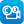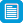Search results

Refine by topic

Main topic Specific topic
Related topic
Listed under:  Mathematics  >  Number (Mathematics)  >  DecimalsThis is a video demonstration, with audio commentary, of the procedure of adding decimal fractions. In the commentary, the presenter emphasises the links with the underpinning concepts of place value. Practice questions, limited to addition of decimal fractions involving tenths and hundredths, are presented with answers, ...Multiplication of decimals by a whole number

This is a four-page HTML resource about solving problems concerning the multiplication of decimals. It contains one video and four questions, two of which are interactive. The resource discusses and explains solving problems involving multiplying a decimal by a whole number to reinforce the students' understanding.Decimals and fractions

This is a video demonstration of converting decimal fractions to simplified fractions. In the commentary, the presenter highlights the relevance of understanding and applying place value concepts, including the efficiency in initially interpreting the fractional part of the decimal fraction as a single fraction. Practice ...reSolve: Authentic Problems: 10000 Centicubes

This sequence of four lessons integrates content in number and measurement to deepen students' understanding and confidence working with larger numbers. Students work flexibly with numbers up to 10 000 as they determine suitable dimensions for a container that can hold 10 000 centicubes. They are challenged to plan, construct ...Rounding decimals to the nearest tenth

This is a digital resource comprising a video demonstration of rounding a decimal fraction to the nearest tenth. The commentary uses and interchanges between the language of decimal places and decimal place values expressed in terms of tenths and hundredths. Practice questions dealing more broadly with rounding numbers ...Rounding decimals

This is a six-page HTML resource about solving problems concerning rounding. It contains one video and eight questions, four of which are interactive. The resource discusses and explains solving problems with rounding of decimals to reinforce students' understanding.Subtracting decimals

This is a video demonstration, with audio commentary, of the procedure for pen-and-paper subtraction of decimal fractions. In the commentary, the presenter emphasises the links with the underpinning concepts of place value. Practice questions, limited to subtraction of decimal fractions involving tenths and hundredths, ...reSolve: Modelling Motion - Year 7

This sequence of seven lessons challenges students to use simple equipment to predict, observe and represent motion. They create a series of graphs to represent motion and construct instruments to measure forces in one and then two dimensions. They interpret these representations to develop concepts of force and motion. ...Converting decimals to percentages

This interactive resource consists of a series of questions about converting decimals into percentages. The viewer is required to convert a decimal number into a percentage and enter the answer in a window on the screen. The answer can be checked and, if correct, the viewer moves to the next question. Help is available ...Imperial pie

This activity involves making a cake using a recipe in which the quantities of the ingredients required are measured using a variety of imperial units. To complete the recipe, students need to convert the imperial units to metric units in order to be able to use their metric measuring instruments. The activity serves to ...Connecting fractions, decimals and percentages

This is a website designed for both teachers and students that addresses the connections between fractions, decimals and percentages from the Australian Curriculum for year 6 students. It contains material on the relationship between fractions, decimals and percentages and helps students understand that although there are ...Rounding decimals

This is a website designed for both teachers and students that addresses rounding of decimal numbers from the Australian Curriculum for year 7 students. It contains material on writing a decimal correct to a number of decimal places and rounding money amounts to the nearest five cents. There are pages for both teachers ...The Numberline

An interactive tool that can help students explore a number line, including points representing integers, fractions and decimalsMoneySmart: How much love can fit into a shoebox?

This is a year 4 mathematics unit of work about preparing a 'shoebox of love', a gift-filled shoebox to be sent to a child in need. The unit is intended to take about 11 hours of teaching and learning time. It consists of an introduction, seven student activities and teacher notes on curriculum, pedagogy and assessment. ...MoneySmart: It's raining cats and dogs...and chickens?

This is a year 6 mathematics unit of work about keeping pets. The unit is intended to take about 12 hours of teaching and learning time, and is recommended for near the end of the school year. It consists of an introduction, seven sets of student activities, and teacher notes. The student activities include building a word ...reSolve: Reconciliation data

This lesson prompts students to examine data from the Reconciliation Barometer report, collect their own local data and compare with the national findings. Students discuss the meaning of reconciliation, explore statements from Reconciliation Barometer report, design a survey to collect local data relating to the statements ...TIMES Module 18: Number and Algebra: decimals and percentages - teacher guide

This is a 21-page guide for teachers containing an introduction to decimals and percentages. It shows how place value is extended to describe positive numbers less than one. It also explores how to represent decimals on the number line, compare decimals, undertake the four basic arithmetic operations with decimals and change ...Multiplication and division of decimals

This is a website designed for both teachers and students that addresses multiplication and division of decimals from the Australian Curriculum for year 7 students. It contains material on multiplication and division of decimals and helps students to understand multiplication and division as inverses of each other. There ...Ordering Decimals

An animated tutorial about ordering numbers with up to 3 decimal places. An interactive quiz is included.Percentage discounts

This is a website designed for both teachers and students that refers to making connections between percentages, decimals and fractions, as well as calculating percentages and percentage discounts from the Australian Curriculum for year 6 students. It contains material on calculating percentages of an amount and calculating ...